Nonequispaced fast Fourier transform
Nonequispaced DCT/DST

# Fast Trigonometric Transforms For Non-Equispaced Nodes

In many areas computers are increasingly used. Thus, the need for fast algorithms in fundamental tasks is constantly growing. One of the most frequent problems are certainly transformations such as DFT (discrete fourier transform), DCT (discrete cosine transform), and DST (discrete sine transform). Fast algorithms have been known for a longer period of time now, however, they all come with the same restriction. The nodes have to be on an equispaced grid. Today, as a matter of fact, there is an increasing number of applications working with non-uniform sampling sets. To allow for this trend, we propose fast algorithms and provide an implementation.

We consider the index set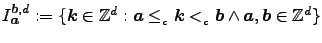, where inequalities hold componentwise. Furthermore, let the pointwise product of two vectors v = k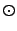x be v := (k0x0, k1x1, ..., kd-1xd-1)T. We define the cos and sin of a vector v as the tensorproduct of its components, cos(v) := cos(v0)cos(v1)...cos(vd-1) and sin(v) := sin(v0)sin(v1)...sin(vd-1), respectively.
Let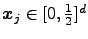, (j = 0, ..., M-1),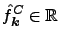,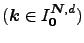, and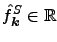,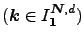then the d-variate NDCT and NDST are defined as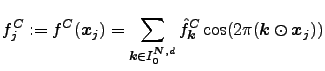and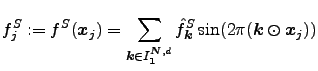respectively.

The libraries are written in C, containing the NFCT and NFST as well as their inverse transforms iNFCT and iNFST.

## Example

Given a set of M arbitrary pointsin d-dimensional space and samples f(xj ), we aim to find the coefficients of the d-variate trigonometric polynomial g(xj ) that suffices f(xj ) ≈ g(xj ). By using an iterative equation system solver in combination with the NFCT or NFST algorithms we can accomplish this problem known as Scattered Data Interpolation. We used the glacier data set, as an example for the problem stated above: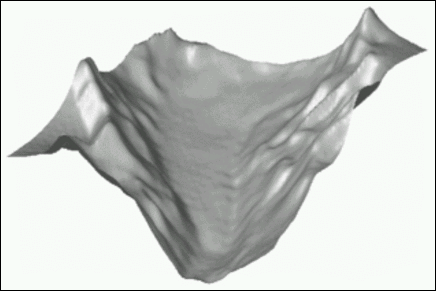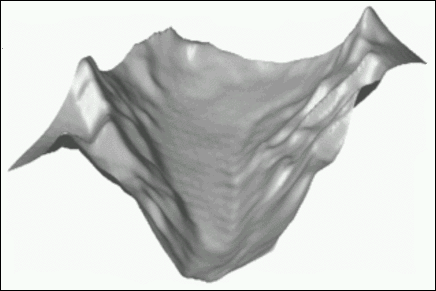Figure 1: These images are the results of two-dimensional scattered data interpolations employing the iterative solver CGNR that uses the NFCT (left) and NFST (right), respectively, for fast matrix-vector multiplication.

## Runtime

May N = (N0, ..., Nd-1)T be the bandwidths, M the number of nodes x, d the dimension and m the truncation parameter. Then, our fast algorithms (NFCT and NFST) have a complexity of O((N)log∏(N) + mdM) while the slow direct summations perform the transform in O((N)M) arithmetic operations, where ∏(N) := N0N1...Nd-1.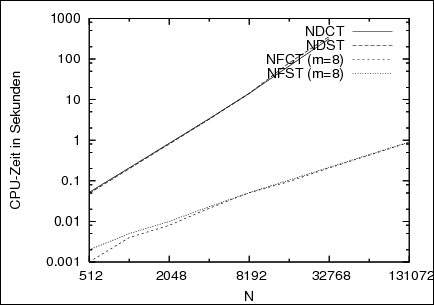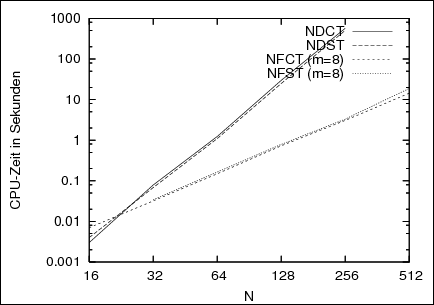Figure 2: These graphs show running times (in seconds) of the one-dimensional (left) and two-dimensional (right) NFCT and NFST in respect to N for random Fourier coefficients and nodes. Times are compared to the direct summation (NDCT/NDST).

## Approximation Error

The NFCT and NFST are approximate algorithms. Consequently, we make an approximation error E(xj ), that we estimate with E(xj ) as the maximum of the sum of the aliasing error |Ea(xj )| (cutting in frequency domain) and the truncation error |Et(xj )| (cutting in spatial domain), (j=0,...,M-1). The locality of the kernel (i.e. Gauss, B-Spline, Sinc or Kaiser-Bessel) that is used in both spatial and frequency domain, plays a significant role concerning the accuray of the results. With the truncation parameter m and the oversampling-factor σ, we can conveniently control the outcome, possibly at the cost of runtime.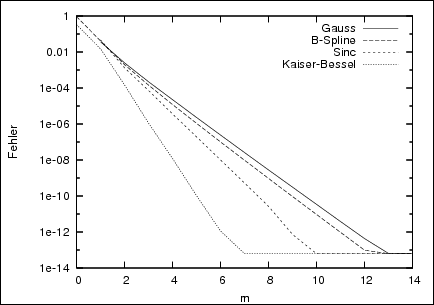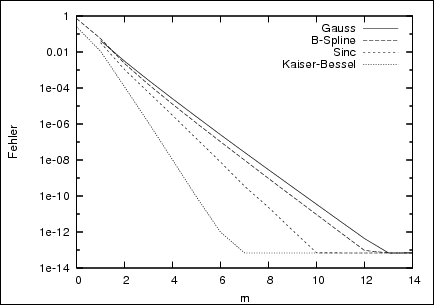Figure 3: These graphs show the approximation error E∞ of the NFCT (left) and NFST (right) in respect to m and various kernels for the dimension d=1.

## Usage

Two simple examples how to use the NFCT/NFST and iNFCT/iNFST are shown below ("R" is used as a wildcard for "c" and "s"):
```
void my_2d_nfRt_trafo();
{
int j,k;
nfRt_plan my_plan;

// initializing plan for two dimensional transform
// with bandwitdhs 32, 32 and 1024 nodes
nfRt_init_2d( &my_plan, 32, 32, 1024);

// initializing the nodes
for( j = 0;  j < my_plan.d * my_plan.M_total;  j++)
my_plan.x[j]  = 0.5 * ((double)rand()) / RAND_MAX;

// if PRE_PSI is set, then do precomputation
if( my_plan.nfRt_flags & PRE_PSI)
nfRt_precompute_psi( &my_plan);

// initializing coefficients
for( k = 0;  k < my_plan.N_total;  k++)
my_plan.f_hat[k] = ((double)rand()) / RAND_MAX;

// execute transform
nfRt_trafo( &my_plan);

// process results (i.e. print to stdout)
for( j = 0; j <  my_plan.M_total;  j++)
printf( "f[%d] = %e\n", j, my_plan.f[j]);

// deallocate memory
nfRt_finalize( &my_plan);
}
```

```
void my_2d_inv_nfRt();
{
int j,k;
int iter, iter_end = 5;
nfRt_plan  my_plan;
infRt_plan my_inv_plan;

// initializing plan for two dimensional transform
// with bandwidths 32, 32 and 1024 nodes
nfRt_init_2d( &my_plan, 32, 32, 1024);

// initializing plan for an inverse transform
infRt_init( &my_inv_plan, &my_plan);

// initializing nodes
for( j = 0; j < my_plan.d * my_plan.M_total;  j++)
my_plan.x[j]  = 0.5 * ((double)rand()) / RAND_MAX;

// if PRE_PSI is set, then do precomputation
if( my_plan.nfRt_flags & PRE_PSI)
nfRt_precompute_psi( &my_plan);

// initializing samples
for( j = 0; j < my_plan.M_total; j++)
my_inv_plan.y[j] = ((double)rand()) / RAND_MAX;

// initializing fourier coefficients
for( k = 0; k < my_plan.N_total;  k++)
my_inv_plan.f_hat_iter[k] = 0.0;

// precomputations for the iterative solver
infRt_before_loop( &my_inv_plan);

// execute iterations
for( iter = 0;  iter < iter_end;  iter++)
infRt_loop_one_step( &my_inv_plan);

// further processing  (i.e. print to stdout)
for( k = 0;  k < my_plan.N_total;  k++)
printf( "%e\n", my_inv_plan.f_hat_iter[k]);

// deallocate memory
infRt_finalize( &my_inv_plan);
nfRt_finalize( &my_plan);
}
```

Steffen Klatt, Nov. 2005

The algorithms are implemented by Steffen Klatt in ./examples/nfst and ./examples/nfct. Related paper are
• Fenn, M. and Potts, D.
Fast summation based on fast trigonometric transforms at nonequispaced nodes.
Numer. Linear Algebra Appl. 12, 161-169. (full paper ps, pdf),   2005